Next: Spherical Harmonics Up: Orbital Angular Momentum Previous: Eigenvalues of

# Eigenvalues of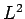Consider the angular wavefunction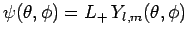. We know that(576)

sinceis a positive-definite real quantity. Hence, making use of Eqs. (194) and (539), we find that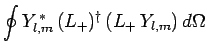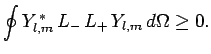(577)

It follows from Eqs. (541), and (556)-(558) that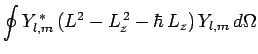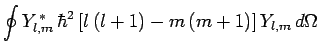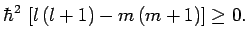(578)

We, thus, obtain the constraint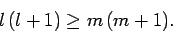(579)

Likewise, the inequality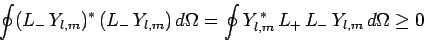(580)(581)

Without loss of generality, we can assume that. This is reasonable, from a physical standpoint, since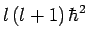is supposed to represent the magnitude squared of something, and should, therefore, only take non-negative values. If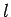is non-negative then the constraints (579) and (581) are equivalent to the following constraint: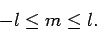(582)

We, thus, conclude that the quantum numbercan only take a restricted range of integer values.

Well, ifcan only take a restricted range of integer values then there must exist a lowest possible value it can take. Let us call this special value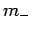, and let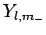be the corresponding eigenstate. Suppose we act on this eigenstate with the lowering operator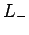. According to Eq. (560), this will have the effect of converting the eigenstate into that of a state with a lower value of. However, no such state exists. A non-existent state is represented in quantum mechanics by the null wavefunction,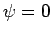. Thus, we must have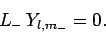(583)

Now, from Eq. (540),(584)

Hence,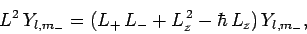(585)

or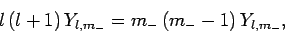(586)

where use has been made of (556), (557), and (583). It follows that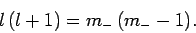(587)

Assuming thatis negative, the solution to the above equation is(588)

We can similarly show that the largest possible value ofis(589)

The above two results imply thatis an integer, sinceandare both constrained to be integers.

We can now formulate the rules which determine the allowed values of the quantum numbersand. The quantum numbertakes the non-negative integer values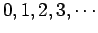. Onceis given, the quantum numbercan take any integer value in the range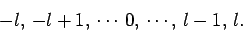(590)

Thus, if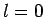thencan only take the value, if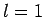thencan take the values, if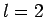thencan take the values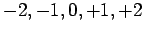, and so on.Next: Spherical Harmonics Up: Orbital Angular Momentum Previous: Eigenvalues of
Richard Fitzpatrick 2010-07-20